# Difference between revisions of "User:Trefor"

Announcements go here

## Typed Notes

The notes below are by the students and for the students. Hopefully they are useful, but they come with no guarantee of any kind.

### First Hour

Recall we had defined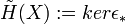$\tilde{H}(X):= ker\epsilon_*$ where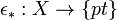$\epsilon_*:X\rightarrow\{pt\}$,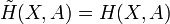$\tilde{H}(X,A) = H(X,A)$

For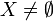$X\neq\empty$,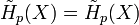$\tilde{H}_p(X) = \tilde{H}_p(X)$ for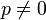$p\neq 0$ and equals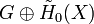$G\oplus\tilde{H}_0(X)$ for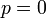$p=0$

This homology definition satisfies the axioms with the following changes: Exactness only for$X\neq\empty$ and the dimension axiom being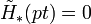$\tilde{H}_*(pt) = 0$. Furthermore, instead of additivity we have, under mild conditions of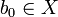$b_0\in X$ and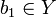$b_1\in Y$ (ie non empty) define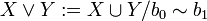$X\vee Y:= X\cup Y / b_0\sim b_1$ for a disjoint union. Then,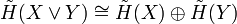$\tilde{H}(X\vee Y)\cong \tilde{H}(X)\oplus \tilde{H}(Y)$

We can actually get the above isomorphism in the following way. There are natural projection maps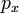$p_x$ and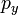$p_y$ from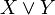$X\vee Y$ to X and Y respectively that simply contract Y and X respectively to the glued base point. There are also natural inclusion maps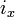$i_x$ and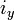$i_y$ going the other way. Then,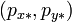$(p_{x*},p_{y*})$ and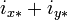$i_{x*}+i_{y*}$ are the two maps in the isomorphism. Proving they are in fact an isomorphism is a homework problem that uses excision to prove it.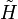$\tilde{H}$ is "kinda" natural:

We have a chain complex where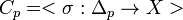$C_p = <\sigma:\Delta_p\rightarrow X>$ where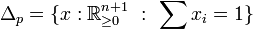$\Delta_p=\{x:\mathbb{R}^{n+1}_{\geq 0}\ :\ \sum x_i = 1\}$

We thus get that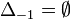$\Delta_{-1} = \empty$ since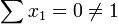$\sum x_1 = 0\neq 1$ vacuously.

Therefore,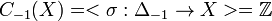$C_{-1}(X) = <\sigma:\Delta_{-1}\rightarrow X> = \mathbb{Z}$

So,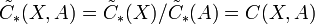$\tilde{C}_*(X,A) = \tilde{C}_*(X)/\tilde{C}_*(A) = C(X,A)$

Note: We have never actually specified that p is positive axiomatically. In fact,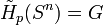$\tilde{H}_p(S^n) = G$ for p=n and 0 for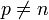$p\neq n$ works fine for all p's. So, since the spaces we are going to be interested in are those that can be constructed from spheres we really will only encounter non trivial homologies for positive p.

Degrees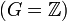$(G=\mathbb{Z})$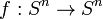$f:S^n\rightarrow S^n$ then get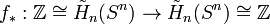$f_*:\mathbb{Z}\cong \tilde{H}_n(S^n)\rightarrow \tilde{H}_n(S^n)\cong\mathbb{Z}$

We thus define: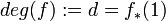$deg(f):=d = f_*(1)$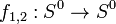$f_{1,2}:S^0\rightarrow S^0$ has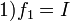$1) f_1 = I$

2)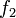$f_2$ = flip, ie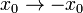$x_0\rightarrow -x_0$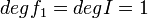$deg f_1 = deg I = 1$ in all dimensions

deg$f_2$ = deg flip = -1

Proposition

Let$f:S^n\rightarrow S^n$ be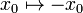$x_0\mapsto-x_0$ and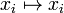$x_i\mapsto x_i$ for i>0

then def f= -1

Proof:

We get two rows of the following sequence, with the induced maps from f going vertically between them: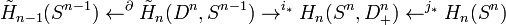$\tilde{H}_{n-1}(S^{n-1})\leftarrow^{\partial}\tilde{H}_n(D^n,S^{n-1})\rightarrow^{i_*} H_n(S^n, D^n_+)\leftarrow^{j_*}H_n(S^n)$

The resulting diagram from the two rows of the above sequence and the maps induced by f between them in fact commute at all places, where the left square commutes as a result of the properties of the connecting homomorphism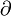$\partial$

Propositions:

1) if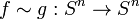$f\sim g: S^n\rightarrow S^n$ then deg f = deg g

2)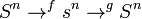$S^n\rightarrow^f s^n\rightarrow^g S^n$ then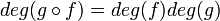$deg (g\circ f) = deg (f) deg (g)$

3) deg a where a is the antipodal map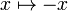$x\mapsto -x$ has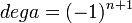$deg a = (-1)^{n+1}$ on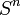$S^n$

### Second Hour

Corollary

If n is even, a is not homotopic to I

Corollary Every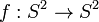$f:S^2\rightarrow S^2$ has a fixed point, or an antipodal point. Ie. f(x) = x or f(x) = -x for some value or x. (Note this is believed true for 2n not just 2, but the follow proof appears needs some modification to work in dimensions 2n)

Proof

Suppose f has no fixed points. Thus x and f(x) are distinct and define a great circle. Thus there is a shortest path from f(x) to -x. This uniquely defines a homotopy between f and a. Suppose f also had no antipodal points. Then the same great circle defines a unique homotopy between f and I. But I is not homotopic to a, a contradiction. Q.E.D

Corollary

Every vector field on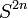$S^{2n}$ has a zero, i.e., "on earth there must be a windless points" or "you can't comb the hair on a coconut"

Proof A non zero vector field induces a homotopy of I to a which is impossible.

Theorem

If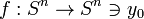$f:S^n\rightarrow S^n \ni y_0$ is smooth (and every map may be approximated by one) and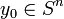$y_0\in S^n$ is a regular value (which occurs almost everywhere by Sard's Theorem) and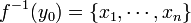$f^{-1}(y_0) = \{x_1,\cdots, x_n\}$ then deg f =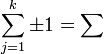$\sum_{j=1}^k \pm 1 = \sum$ sign (det(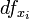$df_{x_i}$))

.ie. we get +1 if it preserves orientation and -1 if it reverses it. The latter term is done using an identification of the coordinates near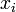$x_i$ with coordinates near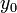$y_0$ using an orientation preserving rotation of$S^n$

Examples

1)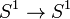$S^1\rightarrow S^1$ via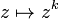$z\mapsto z^k$

This map wraps the circle around itself k times yielding k preimages for each point in the image, all with the same sign.

So,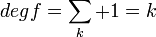$deg f = \sum_k +1 = k$

2) Consider the map of a sphere where you place a plastic bag over a sphere, collect the bag at a pole, twist it once, rewrap the sphere, twist and rewrap again k times. Then the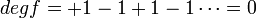$deg f = +1 -1 +1-1\cdots = 0$

Proof of Theorem

1) Let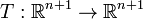$T:\mathbb{R}^{n+1}\rightarrow\mathbb{R}^{n+1}$ be linear and norm preserving,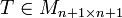$T\in M_{n+1\times n+1}$ and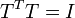$T^{T}T = I$. Then, deg T = det T

Proof: Every rigid rotation is a product of reflections.

2) Let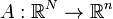$A:\mathbb{R}^N\rightarrow\mathbb{R}^n$ be any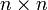$n\times n$ non singular matrix so that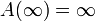$A(\infty)=\infty$ so this induces a map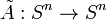$\tilde{A}:S^n\rightarrow S^n$. Then,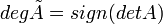$deg\tilde{A} = sign(det A)$

Proof: Gaussian elimination results in making A a product of "elementary matrices" which come in three types: A matrix with 1's along the diagonal except one diagonal entry being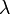$\lambda$. A matrix which is the identity only with two rows interchanged. A matrix which is the identity with a$\lambda$ in some non diagonal location.

The latter of these is clearly homotopic to the identity by simply turning the$\lambda$ off.

The middle of these is just a reflection. The former of these, if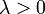$\lambda>0$ it is clearly homotopic to the identity. But if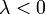$\lambda<0$ then it is homotopic to a reflection.

3)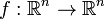$f:\mathbb{R}^n\rightarrow\mathbb{R}^n$ such that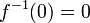$f^{-1}(0) = 0$,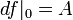$df|_0 = A$ non singular,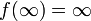$f(\infty) = \infty$ so f defines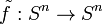$\tilde{f}:S^n\rightarrow S^n$ then deg f = sign(det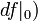$df|_0)$

Proof: Consider for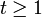$t\geq 1$,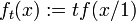$f_t(x) := tf(x/1)$. Then,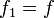$f_1 = f$,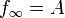$f_{\infty}=A$. This is a homotopy as it makes good sense for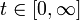$t\in[0,\infty]$. So,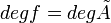$deg f = deg\tilde{A}$

4) All that remains to prove the theorem is the shift from (0,0) to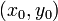$(x_0,y_0)$ which induces a rotation at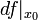$df|_{x_0}$

## Homologies with non trivial negative p's?

In the axiomatic definition of homology there is no specification that the homology groups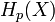$H_p(X)$ must be necessarily trivial for negative p's. That said, in the singular homology that we are developing using CW complexes we only get non trivial homologies for positive p's. The question was raised in class of when you could possibly have non trivial homology at negative p's.

There are infact homology theories that DO have non trivial homology for negative p. In particular, Dr. Putnam from the University of Victoria has defined a homology theory on Smale space and this theory does in fact have non trivial homology groups at negative p's. While Smale spaces have a complicated axiomized definition, very loosely they are a topological space equipped with a metric and a homeomorphism from the space to itself such that locally you can write the space as the direct sum of a section of the space that increases under the homeomorphism(in terms of the metric) and one that decreases. I.e., it is like you have a local coordinate system that tells you where the space is expanding and where it is contracting under the homeomorphism. The classic example of this are Shifts of Finite Type from Dynamical systems. I computed the homology of a particular Smale space consisting of a torus as the space and a particular homeomorphism of it, and found it to have a non trivial homology at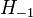$H_{-1}$